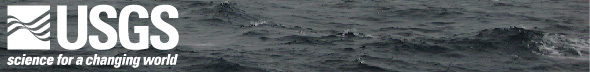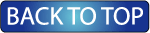Oceanographic Observations, Hudson Shelf Valley, U.S. Geological Survey Open-File Report 02-217

## Tidal Analysis

Amplitude and phase of tidal constituents were computed using the tidal analysis program T_TIDE (Pawlowicz and others, 2002). The principal tidal constituents are K1, O1, M2, N2, and S2. Due to gaps in the data at Station B analysis was only performed for pressure data from January 24, 2000 to April 16, 2000; this short data record prevented analysis for all the tidal constituents.

This report includes summary tables for the principal constituents and the full output from T_TIDE for each station. The T_TIDE output includes the following columns:

• tide: tidal constituent
• freq: frequency (cycles/hour)
• major: major axis of tidal ellipse (for current observations) (cm/s)
• emaj: error estimate for major axis (cm/s)
• minor: minor axis of tidal ellipse (cm/s)
• emin: error estimate for minor axis (cm/s)
• inc: inclination of major axis (counter clockwise from east) (degrees)
• einc: error estimate for inclination (degrees)
• phase: phase of at time of major axis (degrees)
• ephase: error estimate of phase (degrees)
• snr: signal to noise ratio

### Tidal constituents for bottom pressure

Principal tidal constituents (HTML, MSword)

Complete tidal analysis output from T_TIDE (ABCDEF

### Tidal constituents for currents

Principal tidal constituents (HTML, MSword)

Complete tidal analysis output from T_TIDE

### Tidal Ellipses

The tidal current ellipse at each station is plotted in plan view for the ADCP observations obtained at 15 m. The size of the current ellipse is proportional to the current magnitude; the current scale for M2 has been chosen so that the length of the major axis is 5 times the distance a water particle would travel in one-half tidal cycle at map scale (distance traveled = UT/π, where U is the major axis amplitude, and T is the tidal period). The current scale for the small N2, S2, K1, and O1 tidal currents has been chosen so that the length of the major axis is 20 times the distance a water particle would travel in one-half tidal cycle at map scale.

M2 tidal ellipses at 15 m

N2 tidal ellipses at 15 m

S2 tidal ellipses at 15 m

K1 tidal ellipses at 15 m

O1 tidal ellipses at 15 m[an error occurred while processing this directive]